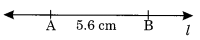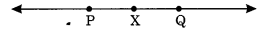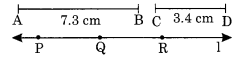# NCERT Solutions For Class 6 Maths Practical Geometry Exercise 14.2

ncert textbook

## NCERT Solutions For Class 6 Maths Practical Geometry Exercise 14.2

NCERT Solutions For Class 6 Maths Chapter 14 Practical Geometry Ex 14.2

Exercise 14.2

Ex 14.2 Class 6 Maths Question 1.
Draw a line segment of length 7.3 cm using ruler.
Solution:
Step I: Mark at point P.
Step II : Place the O mark of the ruler against the point P.
Step III : Mark a point Q at a distance of 7.3 cm from P.
Step IV : Join P and Q.Thus$\overline { PQ }$is the line segment of length 7.3 cm.

Ex 14.2 Class 6 Maths Question 2.
Construct a line segment of length 5.6 cm using ruler and compass.
Solution:
Step I: Draw any line L of suitable lengths.
Step II : Place the needle of the compass on the zero mark of the ruler and open it upto 5.6 mark.
Step III : Place the needle at any point A at the line and draw an arc to cut l at B.Thus,$\overline { AB }$is the required line segment of length 5.6 cm.

Ex 14.2 Class 6 Maths Question 3.
Construct$\overline { AB }$of length 7.8 cm. From this, cut off$\overline { AC }$of length 4.7 cm. Measure$\overline { BC }$.
Solution:
Given that$\overline { AB }$= 7.8 cm and$\overline { AC }$= 4.7 cm.
Step I : Place zero mark of the ruler at A.
Step II : Mark a point B at a distance of 7.8 cm from A.
Step III : Mark another point C at a distance of 4.7 cm from A such that AC = 4.7 cm.
Step IV : On measuring the length of BC, we find that$\overline { BC }$= 3.1 cm.Ex 14.2 Class 6 Maths Question 4.
Given$\overline { AB }$of length 3.9 cm. Construct$\overline { PQ }$such that the length of$\overline { PQ }$is twice that of$\overline { AB }$. Verify by measurement.(Hint : Construct$\overline { PX }$such that the length of$\overline { PX }$= length of$\overline { AB }$then cut off$\overline { XQ }$such that$\overline { XQ }$also has the length of$\overline { AB }$.
Solution:
Step I: Draw a line l of suitable length.
Step II: Draw$\overline { AB }$= 3.9 cm
Step III: From the line, construct$\overline { PX }$=$\overline { AB }$= 3.9 cm.
Step IV: Again construct$\overline { XQ }$=$\overline { AB }$=3.9 cm
Verification:$\overline { PX }$+$\overline { XQ }$=$\overline { AB }$+$\overline { AB }$Thus twice of$\overline { AB }$is equal to$\overline { PQ }$

Ex 14.2 Class 6 Maths Question 5.
Given$\overline { AB }$of length 7.3 cm and$\overline { CD }$of length 3.4 cm, construct a line segment$\overline { XY }$such that the length of XY is equal to the difference between the length of$\overline { AB }$and$\overline { CD }$. Verify the measurement.
Solution:
Step I : Construct$\overline { AB }$= 7.3 cm and$\overline { CD }$= 3.4 cm.Step II: Take a point P on the given line l.
Step III: Construct$\overline { PR }$such that$\overline { PR }$=$\overline { AB }$=$\overline { AB }$= 7.3 cm.
Step IV:Construct$\overline { RQ }$=$\overline { CD }$= 3.4 cm such that PQ =$\overline { AB }$$\overline { CD }$.
Verification : On measuring, we observe that$\overline { PQ }$= 3.9 cm = 7.3 cm 3.4 cm.
=$\overline { AB }$$\overline { CD }$
Thus,$\overline { PQ }$=$\overline { AB }$$\overline { CD }$.